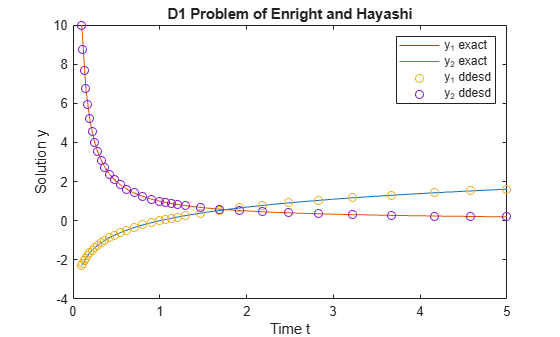# DDE with State-Dependent Delays

This example shows how to use `ddesd` to solve a system of DDEs (delay differential equations) with state-dependent delays. This system of DDEs was used as a test problem by Enright and Hayashi .

The system of equations is

`${{\mathit{y}}_{1}}^{\prime }\left(\mathit{t}\right)={\mathit{y}}_{2}\left(\mathit{t}\right),$`

`${{\mathit{y}}_{2}}^{\prime }\left(\mathit{t}\right)=-{\mathit{y}}_{2}\left({\mathit{e}}^{1-{\mathit{y}}_{2}\left(\mathit{t}\right)}\right)\cdot {\mathit{y}}_{2}{\left(\mathit{t}\right)}^{2}\cdot {\mathit{e}}^{1-{\mathit{y}}_{2}\left(\mathit{t}\right)}.$`

The history functions for $\mathit{t}\le 0.1$ are the analytical solutions

`${\mathit{y}}_{1}\left(\mathit{t}\right)=\mathrm{log}\left(\mathit{t}\right),$`

`${\mathit{y}}_{2}\left(\mathit{t}\right)=\frac{1}{\mathit{t}}.$`

The time delays in the equations are only present in $\mathit{y}$ terms. The delays depend only on the state of the second component ${\mathit{y}}_{2}\left(\mathit{t}\right)$, so the equations form a system of state-dependent delay equations.

To solve this system of equations in MATLAB, you need to code the equations, delays, and history before calling the delay differential equation solver `ddesd`, which is meant for systems with state-dependent delays. You either can include the required functions as local functions at the end of a file (as done here), or save them as separate, named files in a directory on the MATLAB path.

### Code Delays

First, write a function to define the time delays in the system. The only delay present in this system of equations is in the term$-{\mathit{y}}_{2}\left({\mathit{e}}^{1-{\mathit{y}}_{2}\left(\mathit{t}\right)}\right)$.

```function d = dely(t,y) d = exp(1 - y(2)); end ```

Note: All functions are included as local functions at the end of the example.

### Code Equation

Now, create a function to code the equations. This function should have the signature `dydt = ddefun(t,y,Z)`, where:

• `t` is time (independent variable).

• `y` is the solution (dependent variable).

• `Z(n,j)` approximates the delays ${\mathit{y}}_{\mathit{n}}\left(\mathit{d}\left(\mathit{j}\right)\right)$, where the delay $\mathit{d}\left(\mathit{j}\right)$ is given by component `j` of `dely(t,y)`.

These inputs are automatically passed to the function by the solver, but the variable names determine how you code the equations. In this case:

• `Z(2,1)`$\text{\hspace{0.17em}}\to {\mathit{y}}_{2}\left({\mathit{e}}^{1-{\mathit{y}}_{2}\left(\mathit{t}\right)}\right)$

```function dydt = ddefun(t,y,Z) dydt = [y(2); -Z(2,1)*y(2)^2*exp(1 - y(2))]; end ```

### Code Solution History

Next, create a function to define the solution history. The solution history is the solution for times $\mathit{t}\le {\mathit{t}}_{0}$.

```function v = history(t) % history function for t < t0 v = [log(t); 1./t]; end ```

### Solve Equation

Finally, define the interval of integration $\left[{\mathit{t}}_{0}\text{\hspace{0.17em}\hspace{0.17em}}{\mathit{t}}_{\mathit{f}}\right]$ and solve the DDE using the `ddesd` solver.

```tspan = [0.1 5]; sol = ddesd(@ddefun, @dely, @history, tspan);```

### Plot Solution

The solution structure `sol` has the fields `sol.x` and `sol.y` that contain the internal time steps taken by the solver and corresponding solutions at those times. (If you need the solution at specific points, you can use `deval` to evaluate the solution at the specific points.)

Plot the two solution components against time using the history function to calculate the analytical solution within the integration interval for comparison.

```ta = linspace(0.1,5); ya = history(ta); plot(ta,ya,sol.x,sol.y,'o') legend('y_1 exact','y_2 exact','y_1 ddesd','y_2 ddesd') xlabel('Time t') ylabel('Solution y') title('D1 Problem of Enright and Hayashi')```### Local Functions

Listed here are the local helper functions that the DDE solver `ddesd` calls to calculate the solution. Alternatively, you can save these functions as their own files in a directory on the MATLAB path.

```function dydt = ddefun(t,y,Z) % equation being solved dydt = [y(2); -Z(2,1).*y(2)^2.*exp(1 - y(2))]; end %------------------------------------------- function d = dely(t,y) % delay for y d = exp(1 - y(2)); end %------------------------------------------- function v = history(t) % history function for t < t0 v = [log(t); 1./t]; end %-------------------------------------------```

### References

 Enright, W.H. and H. Hayashi. “The Evaluation of Numerical Software for Delay Differential Equations.” In Proceedings of the IFIP TC2/WG2.5 working conference on Quality of numerical software: assessment and enhancement. (R.F. Boisvert, ed.). London, UK: Chapman & Hall, Ltd., pp. 179-193.